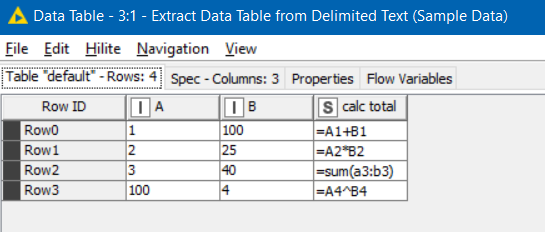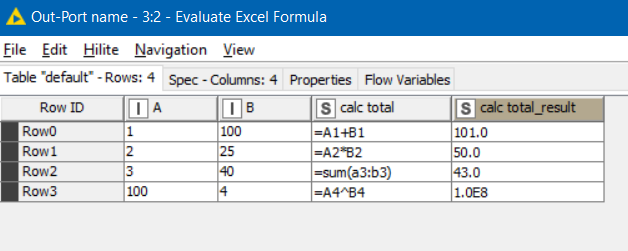# Table Creator

Hi,

I input a formula into table creator so that my workflow would add this to a specific cell.

It has done so. However I would like the output to not be the formula being displayed but the actual value of the formula.

I cannot modify the formula in table creator.

Is there anyway I can modify the format of that formula pre output file ?

any other solution ?

thanks

Hello @jasonk2022 ,

as far as I know, table creator is used to manually input data, not to insert formulas.

If you want to add a new column with a formula, use a column expressions node, a math formula node or a string manipulation node, just to mention some of the main ones.

Have a nice day,
Raffaello

2 Likes

Hi,

I dont need to add new columns or rows.

I require the formula to be placed into a specific cell which I have achieved.

I would simply like to format the cell output so that it doesnt appear as a formula but the actual calculation.

Thanks

Hi @jasonk2022 , as @lelloba rightly says, the Table Creator is for static data.

Given that there is no formula functionality in there, what syntax were you using to write the formulae?

I think if you want advice on how to modify what you are doing, you will need first to actually show us what you are doing…1 Like

Hi,

I input a formula into cell A1 of the table creator eg =(A1+B1)

I have created a workflow whereby that specific formula gets placed into a specific cell of my output spreadsheet. I use the excel updater node to achieve this.

However that output still displays as the actual formula instead of the calculation for A1+B1.

I would like my output to display the actual calculation instead of the formula.

Thanks

Hi @jasonk2022 , this is simply not how KNIME works. Calculations are performed by nodes such as the Math Formula node in the workflow to create the columns based on calculations, or to perform transformations on your data. ”cells" are not referenced in the way you might reference them in Excel . You need to think in terms of columns rather than single cells.

KNIME is a powerful tool but you need to think differently to the way you do something in Excel. Where have you read that you can enter a formula in a cell in this way?

I would suggest that you take a look at some of the basic tutorials to get a feel for how KNIME works. But if you have some sample data and you can show us what end result you want from it, somebody may be able to give you some advice for how this is done.

4 Likes

ok thanks.

I assumed possibly incorrectly that I’d be able to format cells in Knime just like excel. Not only cells but specific cells.

Thanks

As @takbb suggests, have a look at the tutorials. Follow the link below, you’ll get everything you need to get started:

RB

3 Likes

hi @jsonk2022,

There are some “community nodes” which you can install which may help do some of what you are looking for. For example take a look at the AF nodes - a very useful set of utility nodes by @AnotherFraudUser

This one caught my eye in relation to your question:

I hadn’t previously tried it, but given the following input:it generated this:Combined with a column filter to then remove the original formula, and this could be of interest. I would still however recommend learning the actual KNIME nodes way of doing things, but if you really are needing to hand-craft individual cells with different calculations, then this is (maybe) a possible way of achieving it. But keep in mind that there will be limitations to working in this way, and limits you really to working with quite static data.

Have fun!5 Likes

thanks ill take a look that

appreciate the time and effort you have taken to respond

2 Likes

Just a short remark to my node.
Formulars have to be in english and might be quite slow with very large excel files1 Like

This topic was automatically closed 90 days after the last reply. New replies are no longer allowed.